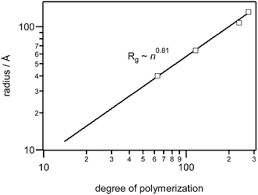## How to Calculate and Solve for Degree of Polymerization | Both Mechanisms | Polymer & TextileThe image above represents degree of polymerization | both mechanisms.

To compute for degree of polymerization, two essential parameters are needed and these parameters are parameter (σ) and parameter (V).

The formula for calculating the degree of polymerization:

Xn = 2V / 1 + σ

Where:

Xn = Degree of Polymerization
σ = Parameter
V = Parameter

Let’s solve an example;
Find the degree of polymerization when the parameter is 12 and the parameter is 18.

This implies that;

σ = Parameter = 12
V = Parameter = 18

Xn = 2V / 1 + σ
Xn = 2(18) / 1 + 12
Xn = 36 / 13
Xn = 2.769

Therefore, the degree of polymerization is 2.769.

Calculating the Parameter when the Degree of Polymerization and Parameter is Given.

V = Xn (1 + σ) / 2

Where:

V = Parameter
σ = Parameter
Xn = Degree of Polymerization

Let’s solve an example;
Find the parameter when the degree of polymerization is 22 and the parameter is 4.

This implies that;

Xn = Degree of Polymerization = 22
σ = Parameter = 4

V = Xn (1 + σ) / 2
V = 22 (1 + 4) / 2
V = 22 (5) / 2
V = 110 / 2
V = 55

Therefore, the parameter is 55.